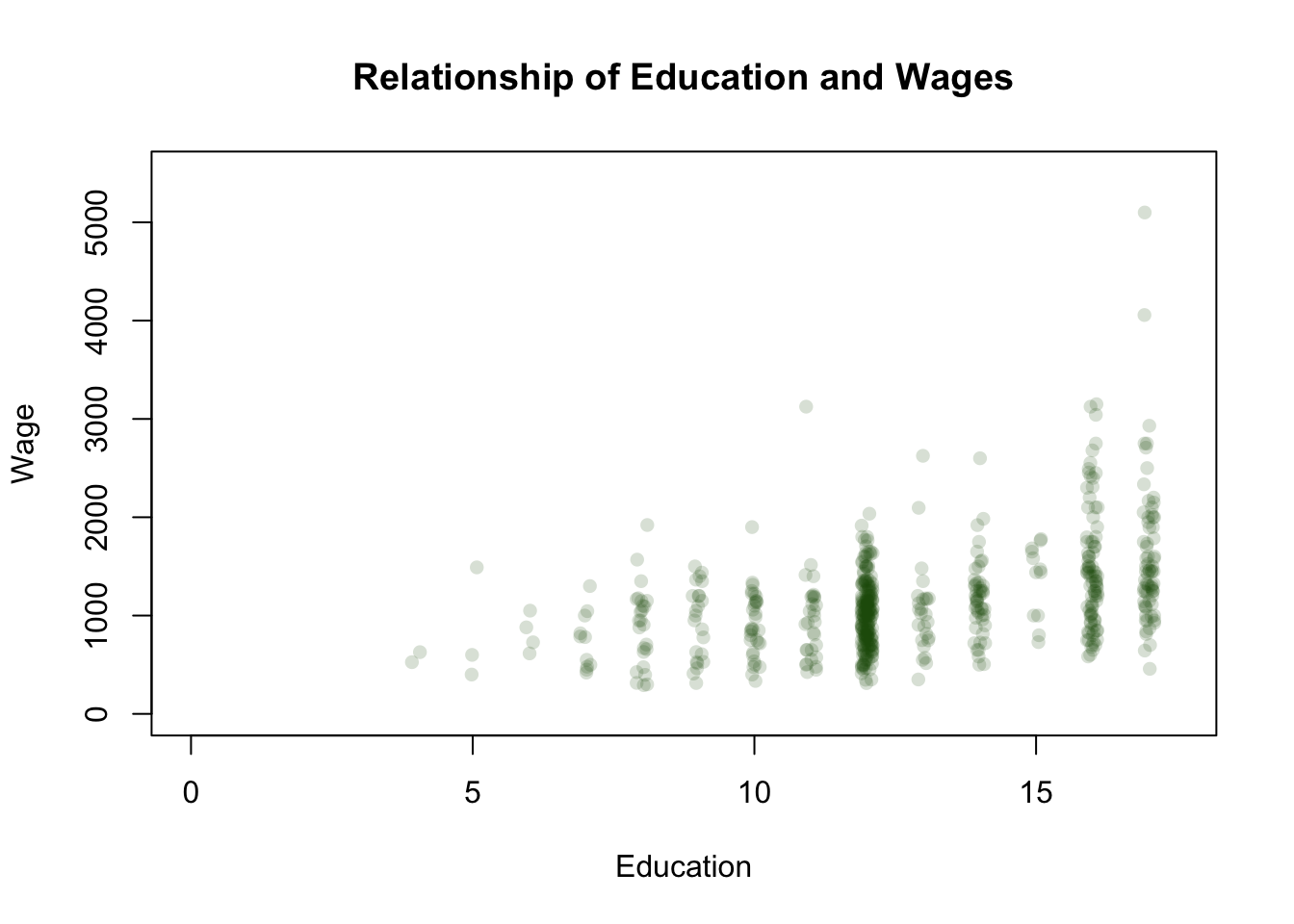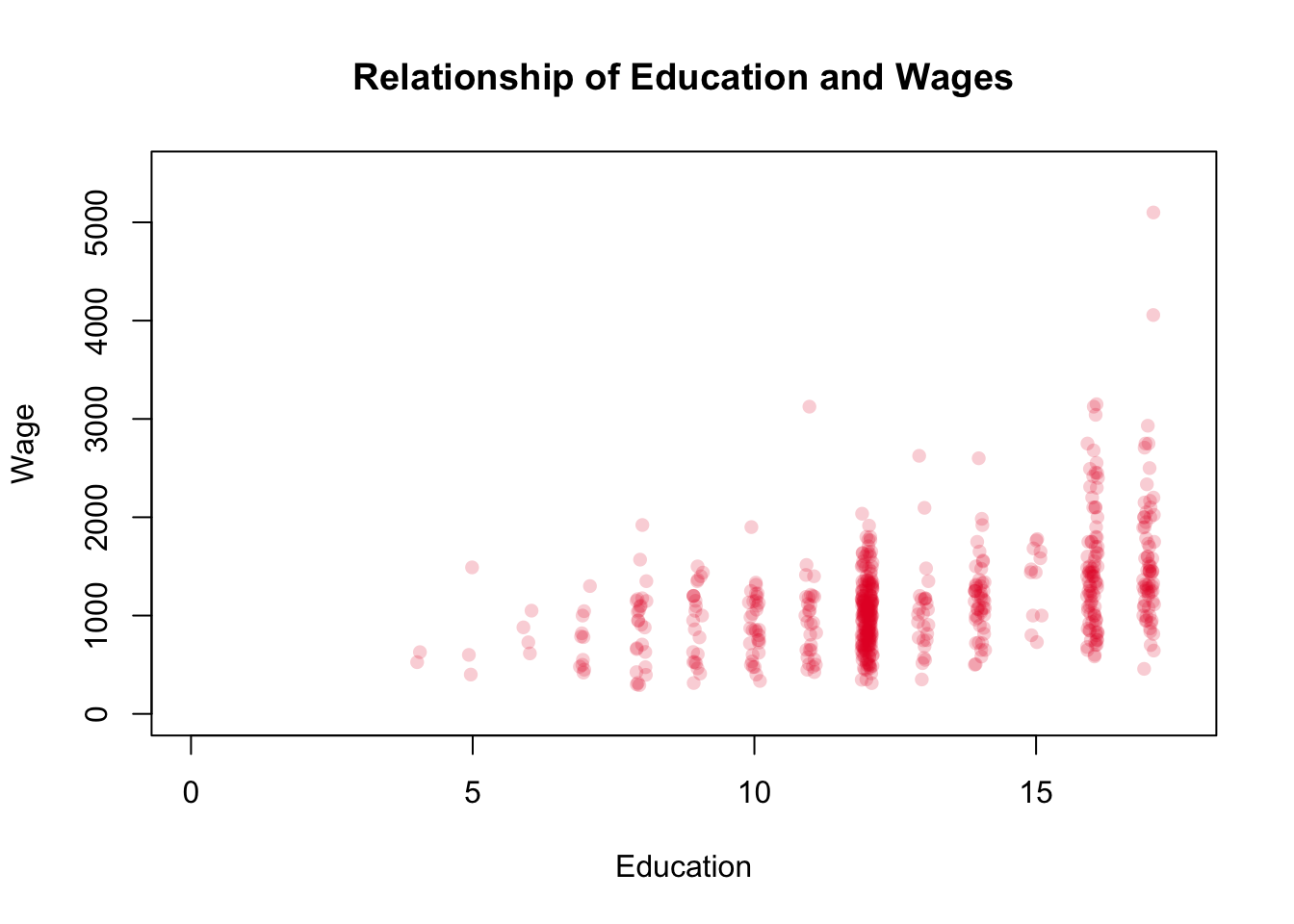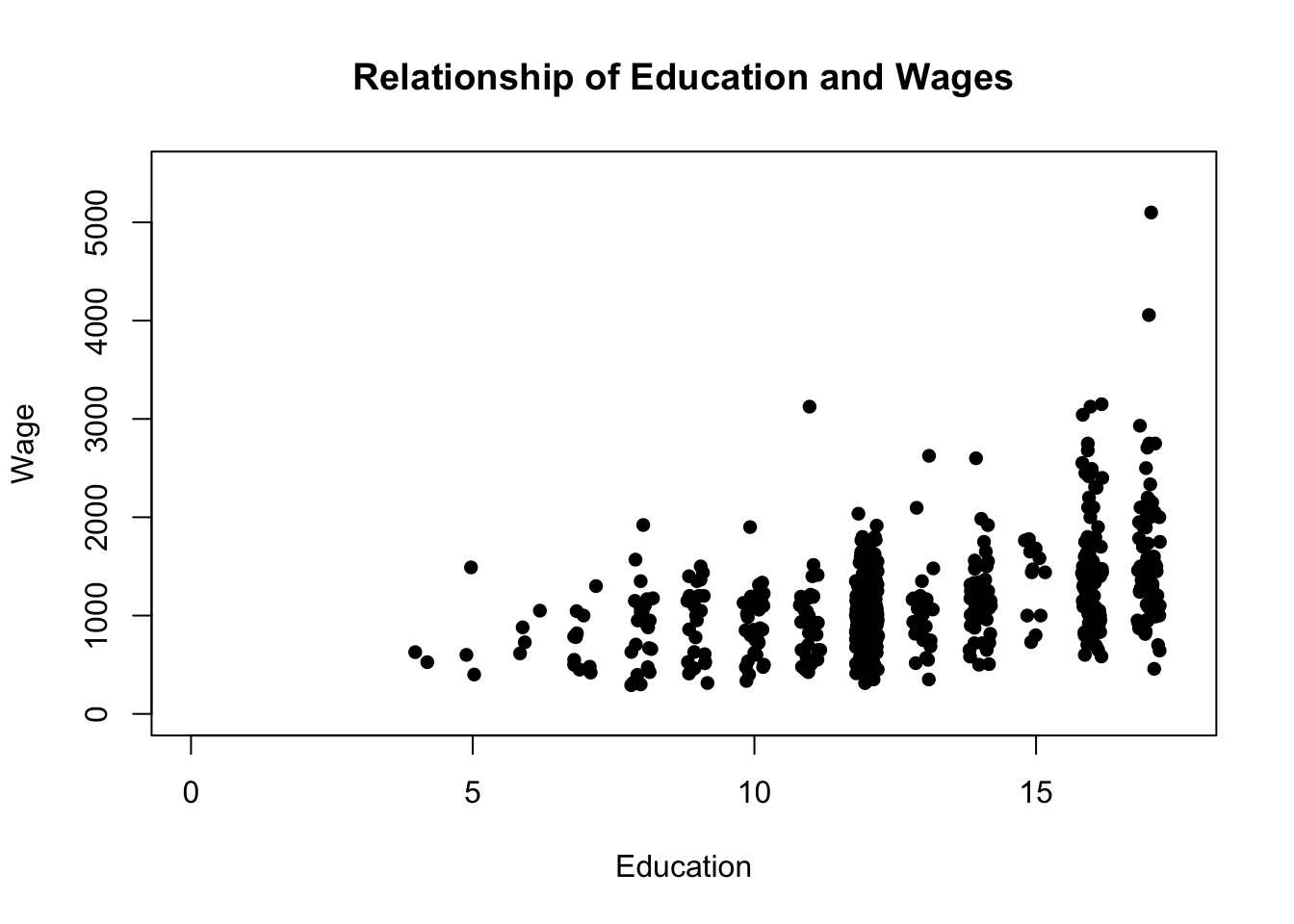# 10 Basic Regression

Understanding t-tests is a significant jump. We can understand if there is a relationship between two variables in the data we have using a correlation. T-tests, and other tools of inference, allow us to see whether that relationship we observe goes beyond the data we have and extends to the population as well.

But there’s a more powerful and flexible tool for understanding the world and specifically making predictions: regression. Regression is a tool that helps us to understand whether drinking milk gives us stronger bones, whether extra years of schooling increases our earnings, or whether red cars are stolen more often. We can begin to answer those question with the tools we already have, but that would be a bit like trying o build a house with only a hammer. Regression gives us a lot more capabilities.

## 10.1 Back to Correlation

Before we move forward, let’s move back. Regression is intimately associated with thing we’ve already learned like correlation and t tests. We’ll first discuss the overlaps in order to orient ourselves, before setting sail into more difficult waters.

At its most basic, regression attempts to find the best fit for a linear relationship between two variables, similarly to what correlation attempted to do. Let’s go back a few chapters and pull up some data we’ve already used.

In the chapter on correlation we used the data on California Schools, and we observed a strong positive correlation between parental income and math scores. We could calculate the correlation coefficient and also plot the data to visualize that relationship.

``````library(AER)
data("CASchools")
cor(CASchools\$income, CASchools\$math)``````
``##  0.6993981``
``````plot(CASchools\$income, CASchools\$math, pch=16,
main="Relationship of Parental Income to Math Scores",
xlab="Parental Income",
ylab="Math Scores")``````We can add an additional detail though. Let’s add a line to the plot to show exactly how strong the relationship is between parental income and math scores.

``````plot(CASchools\$income, CASchools\$math, pch=16,
main="Relationship of Parental Income to Math Scores",
xlab="Parental Income",
ylab="Math Scores")
abline(lm(CASchools\$math~CASchools\$income))``````The upward angle indicates for us that the higher parental income is, the higher we should expect the scores on math tests to be. But how was that line drawn? Why was it drawn right there exactly, and not any lower or higher?

It’s drawn in order to minimize the distance between itself and each point on the plot. There’s no straight line that can run through each point of the scatter plot exactly, so the line is drawn so as to minimize the distance.

The distance between the line and the points are the residuals of the model. We can’t precisely predict a schools math score just by the parental income, so we’re imprecise. And that’s okay, we can make a better prediction using a line of best fit than we can by random.

So similarly to correlation, we’re trying to minimize the residuals and make a better prediction. And regression helps us to make that prediction more precise. The line we drew above is actually a regression line. Let’s reuse that line and begin to identify what we do in regression

It’s worth remembering the equation for a line that you learned back in high school math: y = mx+b.

Y was the y-value on the plot, and in our plot above the y-axis is the school’s math score.

mx is the slope of the line, or the rise over the run. For each one unit increase on the x-axis, how many units do you move up or down on the y-axis. That’s what mx told us. And b is the y-intercept, the point where the line would cross the y-axis. That basic structure underlies regression. Let’s take a closer look at the line of code we used to produce a line above.

``abline(lm(CASchools\$math~CASchools\$income))``

That line of code is made up of 4 components. abline(), lm(), ~, and the two variable names math and income.

abline() is the command to draw a line in the plot, so it’s great if we’re graphing our data but otherwise we don’t need it. Inside of abline() is a function called lm().

lm() stands for linear model. We’re going to create a linear model (meaning a straight line) for the things we put inside that command. Model simply means predict in this case, create a linear prediction of the y value based on the x value.

is essentially the equal sign from the equation for a line we had earlier. We’ll use it as a stand in for an equal sign in a lot of modeling, because the equal sign is used to assign value in r. So as we’re setting up our y=mx+b, the ~ is equivalent to =.

math is on the y-axis of the graph, so it goes where the y is in our equation for a line. B series of elimination then, parental income must be the x, so it goes where the mx is.

To put it all together then, we’re asking r to draw a line on our plot abline() using a straight line lm() that has math as the y-axis and parental income on the x axis. We’re drawing a line predicting the value of math based on the value of parental income.

But what about the b from our equation from a line? Where is the intercept? The intercept is generated by the line, once it’s drawn it can be determined where it crosses the y-axis. And that is part of the output of the regression.

That section may need to be read several times. One more time, for repetition, we’re drawing a straight line to try and predict something on a y-axis (the left side of the ~) using the values of the x-axis (the right side of the ~). So what does regression do for us then? We have our scatter plot, correlation tells us parental income and math are positively related, and we have drawn a line. Ah, but regression tells us how much math scores increase with each one-unit increase in parental income.

## 10.2 Regression Output

We’ll start by reprinting our plot with a colored line, and then we’ll add the full regression output below.

``````plot(CASchools\$income, CASchools\$math, pch=16,
main="Relationship of Parental Income to Math Scores",
xlab="Parental Income",
ylab="Math Scores")
segments(10, 610,  10, (10*1.8+625.53), col="red", lwd=2, lty=3)
segments(20, 610,  20, (20*1.8+625.53), col="red", lwd=2, lty=3)
segments(30, 610,  30, (30*1.8+625.53), col="red", lwd=2, lty=3)
segments(40, 610,  40, (40*1.8+625.53), col="red", lwd=2, lty=3)
segments(50, 610,  50, (50*1.8+625.53), col="red", lwd=2, lty=3)
segments(0, (10*1.8+625.53),  10, (10*1.8+625.53), col="red", lwd=2, lty=3)
segments(0, (20*1.8+625.53),  20, (20*1.8+625.53), col="red", lwd=2, lty=3)
segments(0, (30*1.8+625.53),  30, (30*1.8+625.53), col="red", lwd=2, lty=3)
segments(0, (40*1.8+625.53),  40, (40*1.8+625.53), col="red", lwd=2, lty=3)
segments(0, (50*1.8+625.53),  50, (50*1.8+625.53), col="red", lwd=2, lty=3)

abline(lm(math~income, data=CASchools), col="blue", lty=4, lwd=2)````````summary(lm(math~income, data=CASchools))``
``````##
## Call:
## lm(formula = math ~ income, data = CASchools)
##
## Residuals:
##     Min      1Q  Median      3Q     Max
## -39.045  -8.997   0.308   8.416  34.246
##
## Coefficients:
##              Estimate Std. Error t value Pr(>|t|)
## (Intercept) 625.53948    1.53627  407.18   <2e-16 ***
## income        1.81523    0.09073   20.01   <2e-16 ***
## ---
## Signif. codes:  0 '***' 0.001 '**' 0.01 '*' 0.05 '.' 0.1 ' ' 1
##
## Residual standard error: 13.42 on 418 degrees of freedom
## Multiple R-squared:  0.4892, Adjusted R-squared:  0.4879
## F-statistic: 400.3 on 1 and 418 DF,  p-value: < 2.2e-16``````

The top of the regression output shows us what we’ve calculated, Our y-variable is math scores and the other side of the ~ is parental income.

As we just discussed above residuals is a term for how far off the line is from each observation. Each point has a residual (a perfect match would have a residual of 0) and we want to keep the residuals as small as possible. The largest residual is 34.2, which means some schools math scores was underestimated by 34 points based on the schools income. Another school had its score overestimated by 39 points (the largest negative residual.)

Moving further down the numbers, there are two “coefficients” for this model: the intercept and income. The intercept is the b from our equation for a line. The regression equation figured out a line that would minimize the residuals between each point in the data and a straight line. Once it figured out where that line sat, it figured out where that line would cross the y-axis. In this case, that line would cross the y-axis at 625.53. And you can see that on the plot as well. The line appears to cross the y-axis at 625.

What is the value on the x-axis where the y-axis runs? 0. So 625.53 is the value on the y-variable (math scores) where x=0. So if a school had an average parental income of 0 dollars (very poor) it would be expected to get a math score of 625.53.

So what does the number next to income tell us then? The number is 1.8, which is the slope of our line. For each one-unit increase in parental income (which here is one thousand dollars) we expect math scores to increase by 1.8. So a school with a parental income of one thousand dollars would be expected to get 625.53+1.8 on the statewide math test.

And that’s a linear change. A school that earned \$10k would be expected to earn 10 times 1.8 plus 625.53. We can see that displayed with the vertical red line from 10 that shows where the line predicts the math score of a school with that income, which is at 643.53.

Correlation told us that math scores go up with parental income, regression tells us by how much.

There’s a lot of other numbers on the output from the regression, and we’ll get more comfortable with them over time. Most we should recognize from the last chapter, such as standard errors and t-values. We’re interested in the same exact thing with regressions that we were with t tests. We know that math scores go up with parental incomes in the data we have, but what we’re interested in, what we’re really interested in, is whether that difference was generated by random chance or whether it means that we should believe parental income really has a relationship with math scores.

## 10.3 Statistical Significance

We come back to this phrase, statistical significance. We determined whether our t test was statistically significant by whether the p value was below .05 or not. That’s still a good general rule, but in most regressions we’ll have a bit more to think about. At the far right of the output is our p values, and next to them are stars (or asterisks). The asterisks are there to help us quickly judge whether our results are significant. If a p value is between .1 and .05, so almost significant, it will get a period ‘.’ or sometimes it will get a single star (*).

If the value is between .05 and .01, so more significant but still not a crazy strong finding, it’ll generally get two stars (**).

And if the p value is even smaller, like it is in this analysis, it’ll get the coveted three stars (***) which indicates to readers very quickly that the result is very strong.

|P Value |Strength |Stars | |_____________|_____________|_________| |.1-.05 |Kinda |* or . | |.05-.01 |Stronger |** or * | |.01 or below |Strong |*** or | |.001 or below|Very Strong |* |

R’s default output is actually a bit different with the stars than most programs, which makes it a bit difficult to give you a steadfast rule. In general though, more stars equals stronger results, and anything under .05 is considered significant.

So what about the regression we did above? Yes, the result is very significant. We think there is a very good chance that parental income increases math test scores beyond this sample and there’s a very little we’d get a difference this large by random chance.

## 10.4 A quick summary to this point

### 10.4.1 For running a regression in R

A few rules when you’re running a regression.

Let’s take another look at what we ran earlier, without any of the output. It would probably be wroth copying that line of code and running it in R, and changing the variables to other things that are in the CASchools data set just to get comfortable.

``summary(lm(math~income, data=CASchools))``

If we insert data= and tell the regression what data set we want to use, we can just write the variable labels in the model.

The left side of your equation (or the left side of the ~) is your outcome. What do you want to predict. Above, we predicted math scores. This is called your dependent variable, because it depends on what is on the x axis.

We can run a regression with the command lm() and inserting one variable on the left of a ~ and other variables on the right.

we want to put summary() around the lm() to make the output more readable.

### 10.4.2 For evaluating a regression

Look at the coefficient for the independent variable you tested (not the intercept). If the coefficient is positive, your variable increases the value of your dependent variable. If the coefficient is negative, the independent variable decreases the value of your dependent variable. Both relationships are possible.

Look at the p-value. Is it less than .05? It’s an imperfect rule, but if it’s less than .05 then that is a strong relationship. If it’s higher, than we’re less certain that the relationship you see in the regression wasn’t just generated by chance.

## 10.5 Wrapping Up

Let’s just run a bunch of regressions and quickly see what we learn about math scores. We’re going to keep the dependent variable the same, and change the independent variable.

``summary(lm(math~students, data=CASchools))``
``````##
## Call:
## lm(formula = math ~ students, data = CASchools)
##
## Residuals:
##     Min      1Q  Median      3Q     Max
## -49.267 -13.578  -1.218  13.231  56.739
##
## Coefficients:
##               Estimate Std. Error t value Pr(>|t|)
## (Intercept)  6.547e+02  1.097e+00 596.653   <2e-16 ***
## students    -5.315e-04  2.330e-04  -2.281    0.023 *
## ---
## Signif. codes:  0 '***' 0.001 '**' 0.01 '*' 0.05 '.' 0.1 ' ' 1
##
## Residual standard error: 18.66 on 418 degrees of freedom
## Multiple R-squared:  0.0123, Adjusted R-squared:  0.009933
## F-statistic: 5.204 on 1 and 418 DF,  p-value: 0.02304``````

The more students there are at a school, the lower that math scores are in general. Each additional student decreases math scores by about .0005 points, so if a school had 10,000 more students it could expect scores to be about 5 points lower. That result is somewhat strong, with a p value between .05 and .01, hence the one star by the p value.

``summary(lm(math~computer, data=CASchools))``
``````##
## Call:
## lm(formula = math ~ computer, data = CASchools)
##
## Residuals:
##     Min      1Q  Median      3Q     Max
## -48.332 -14.276  -0.813  12.845  56.742
##
## Coefficients:
##               Estimate Std. Error t value Pr(>|t|)
## (Intercept) 653.767406   1.111619 588.122   <2e-16 ***
## computer     -0.001400   0.002077  -0.674    0.501
## ---
## Signif. codes:  0 '***' 0.001 '**' 0.01 '*' 0.05 '.' 0.1 ' ' 1
##
## Residual standard error: 18.77 on 418 degrees of freedom
## Multiple R-squared:  0.001086,   Adjusted R-squared:  -0.001304
## F-statistic: 0.4543 on 1 and 418 DF,  p-value: 0.5007``````

The number of computers at a school has a small negative effect on math scores in the sample. However, that relationship is not significant, so the effect might not be the same outside of this sample.

``summary(lm(math~expenditure, data=CASchools))``
``````##
## Call:
## lm(formula = math ~ expenditure, data = CASchools)
##
## Residuals:
##     Min      1Q  Median      3Q     Max
## -49.170 -13.474  -0.556  12.747  54.190
##
## Coefficients:
##              Estimate Std. Error t value Pr(>|t|)
## (Intercept) 6.290e+02  7.648e+00  82.243  < 2e-16 ***
## expenditure 4.585e-03  1.430e-03   3.208  0.00144 **
## ---
## Signif. codes:  0 '***' 0.001 '**' 0.01 '*' 0.05 '.' 0.1 ' ' 1
##
## Residual standard error: 18.55 on 418 degrees of freedom
## Multiple R-squared:  0.02402,    Adjusted R-squared:  0.02169
## F-statistic: 10.29 on 1 and 418 DF,  p-value: 0.001442``````

Spending at schools has a positive relationship with math scores. Schools that spend more get higher grades. A school with 1000 more in spending could expect to score roughly 4 points higher on the math tests. That effect is highly significant as noted by the two stars.

## 10.6 A brief note about causality

Many of the same caveats about causality we discussed with correlation apply here. We haven’t proven anything causes math scores to go up. All we’ve found is the relationship between different things and whether those relationships were generated by chance or not. Causality takes more to prove.

## 10.7 Coming Attractions

So a lot of things have a relationship with the average math score at a school apparently. But we can only see the correlation between two variables at once and we can only test the relationships between two at a time with a t test.

That’s where the power of regression comes in. We can throw a lot into a soup and see what comes out. That’ll be the subject of the next chapter.